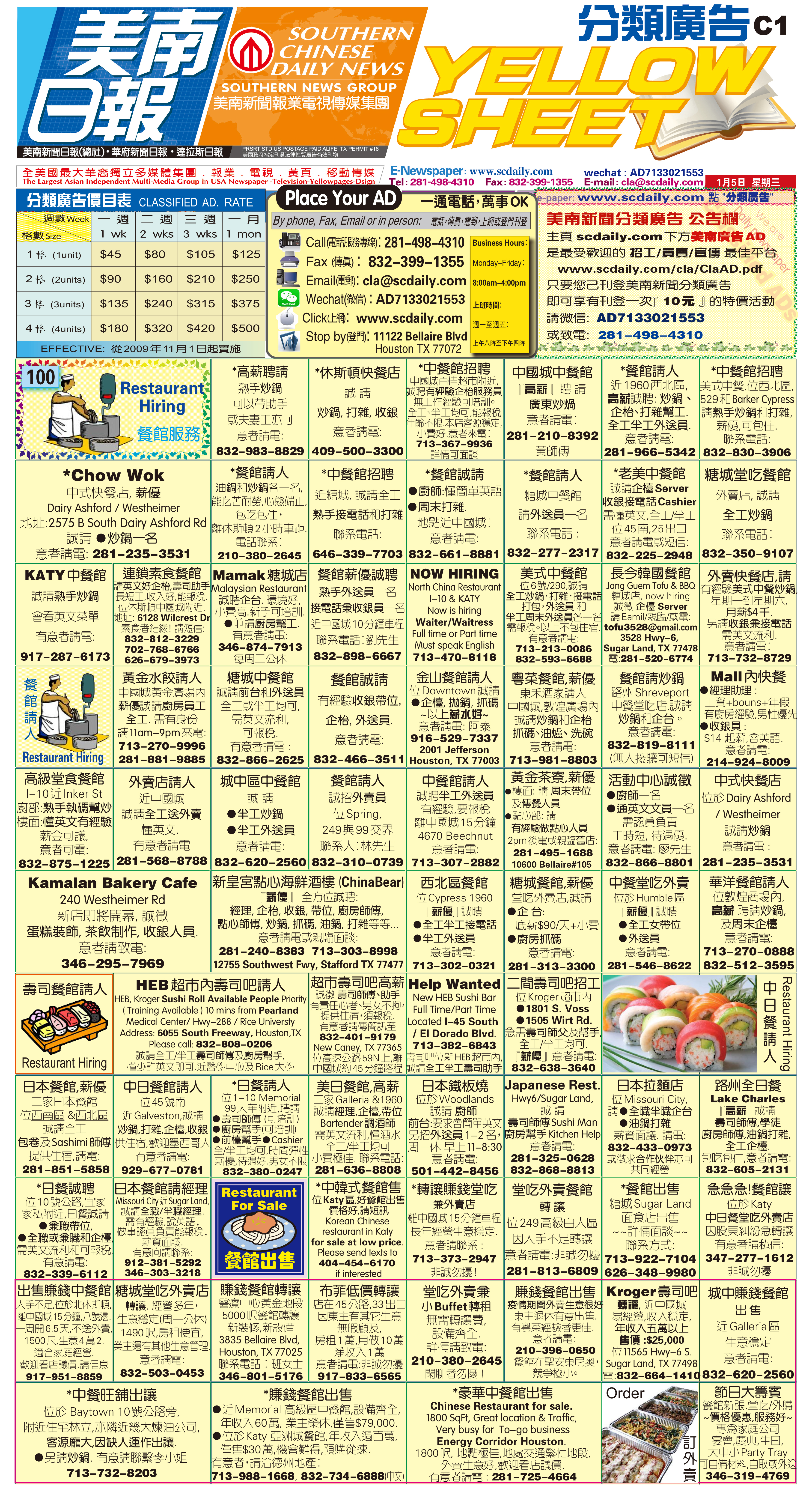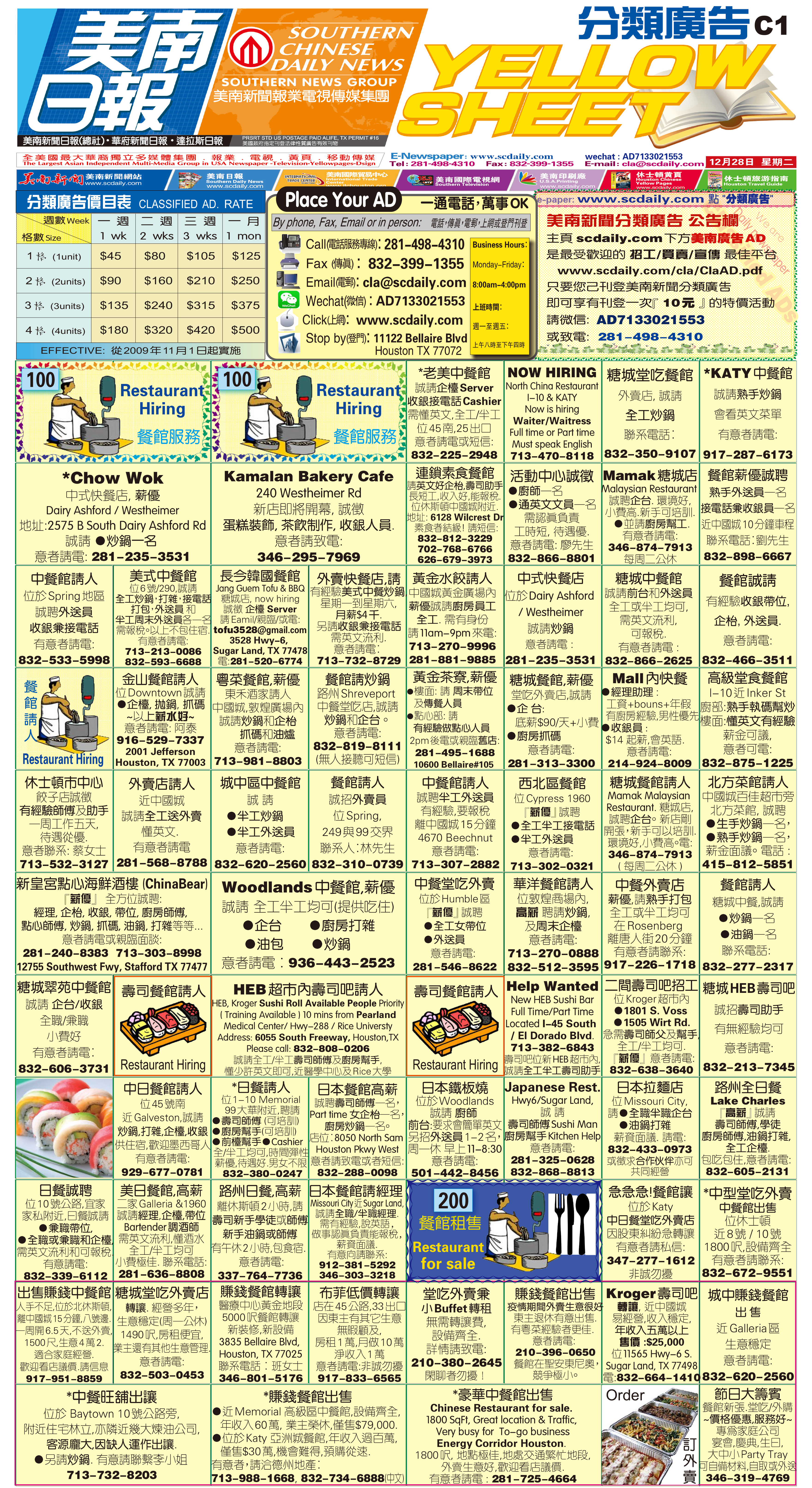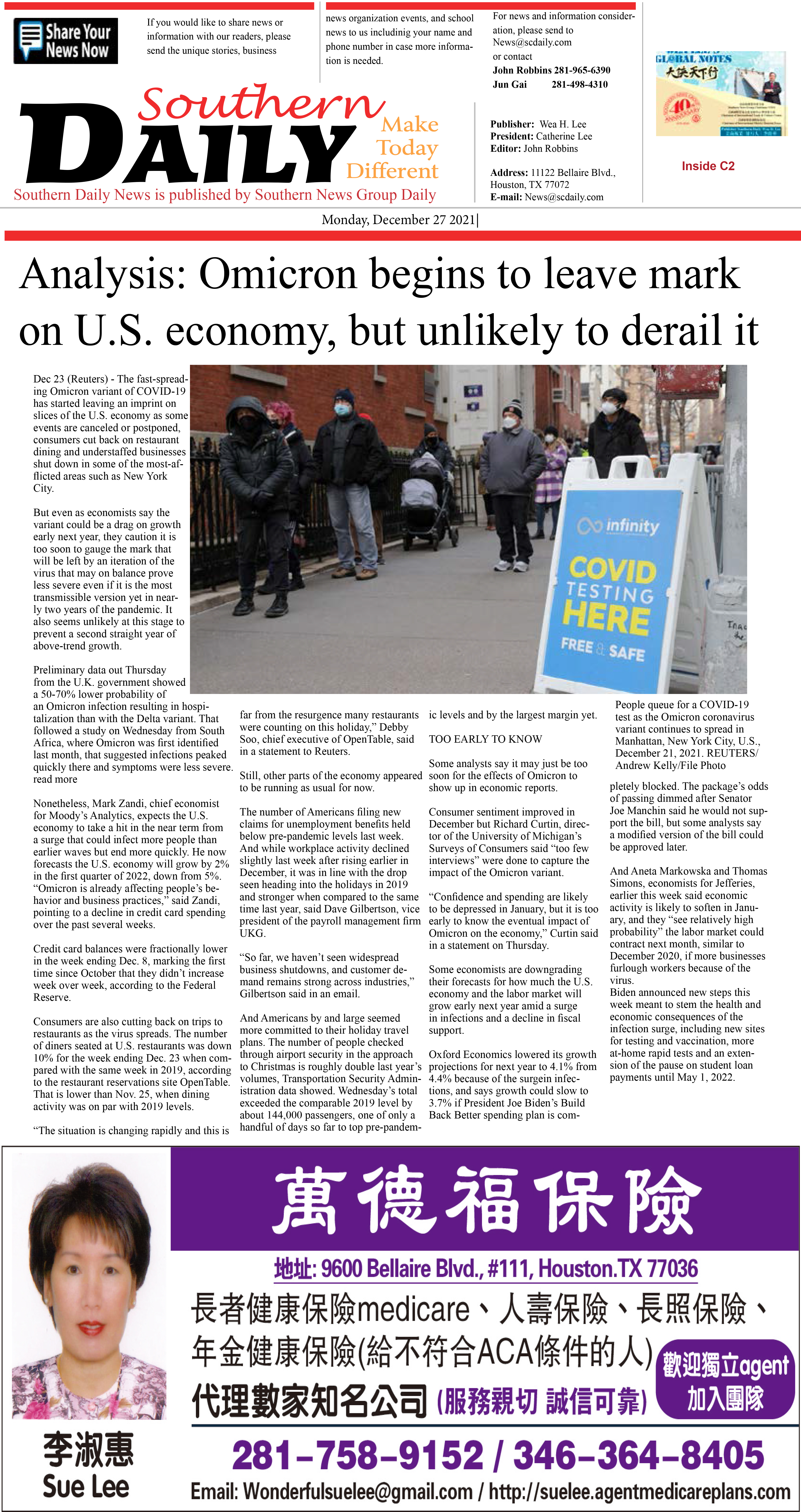220105 Epaper

 A Section B SectionC Section D Section211231 Epaper

 A Section B SectionC Section D Section220104 Epaper

 A Section B SectionC Section D Section220103 Epaper

 A Section B SectionC Section D Section220102 Epaper

 A Section B SectionC Section D Section220101 Epaper

 A Section B SectionC Section D Section211230 Epaper

 A Section B SectionC Section D Section211229 Epaper

 A Section B SectionC Section D Section211228 Epaper

 A Section B SectionC Section D Section211225 Epaper

 A Section B SectionC Section D Section211227 Epaper

 A Section B SectionC Section D Section